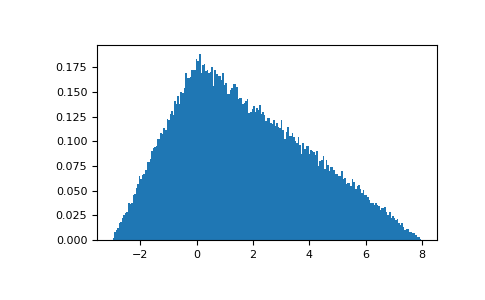# numpy.random.Generator.triangular¶

method

Generator.triangular(left, mode, right, size=None)

Draw samples from the triangular distribution over the interval [left, right].

The triangular distribution is a continuous probability distribution with lower limit left, peak at mode, and upper limit right. Unlike the other distributions, these parameters directly define the shape of the pdf.

Parameters: left : float or array_like of floats Lower limit. mode : float or array_like of floats The value where the peak of the distribution occurs. The value must fulfill the condition left <= mode <= right. right : float or array_like of floats Upper limit, must be larger than left. size : int or tuple of ints, optional Output shape. If the given shape is, e.g., (m, n, k), then m * n * k samples are drawn. If size is None (default), a single value is returned if left, mode, and right are all scalars. Otherwise, np.broadcast(left, mode, right).size samples are drawn. out : ndarray or scalar Drawn samples from the parameterized triangular distribution.

Notes

The probability density function for the triangular distribution isThe triangular distribution is often used in ill-defined problems where the underlying distribution is not known, but some knowledge of the limits and mode exists. Often it is used in simulations.

References

  Wikipedia, “Triangular distribution” https://en.wikipedia.org/wiki/Triangular_distribution

Examples

Draw values from the distribution and plot the histogram:

>>> import matplotlib.pyplot as plt
>>> h = plt.hist(np.random.default_rng().triangular(-3, 0, 8, 100000), bins=200,
...              density=True)
>>> plt.show()#### Previous topic

numpy.random.Generator.standard_t

#### Next topic

numpy.random.Generator.uniform